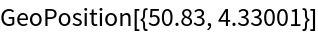#Function Repository Resource:

# FromRDCoordinates

Convert Dutch RD coordinates to GeoPosition objects

Contributed by: Sander Huisman
 ResourceFunction["FromRDCoordinates"][{x,y}] converts the Dutch RD coordinates {x,y} to GeoPosition objects.

## Details and Options

Converts the Dutch RD coordinates (Rijksdriehoekscoördinaten) {x,y} to a latitude-longitude pair in the WGS84 datum using a fourth-order conformal mapping obtained from 6000 references points in the Netherlands.

## Examples

### Basic Examples

Convert an RD coordinate to a GeoPosition object:

 In:=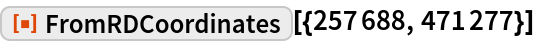Out=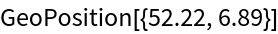### Applications

Plot the waterways of the Netherlands using data from www.pdok.nl given in RD coordinates:

 In:=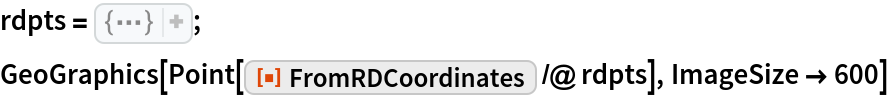Out=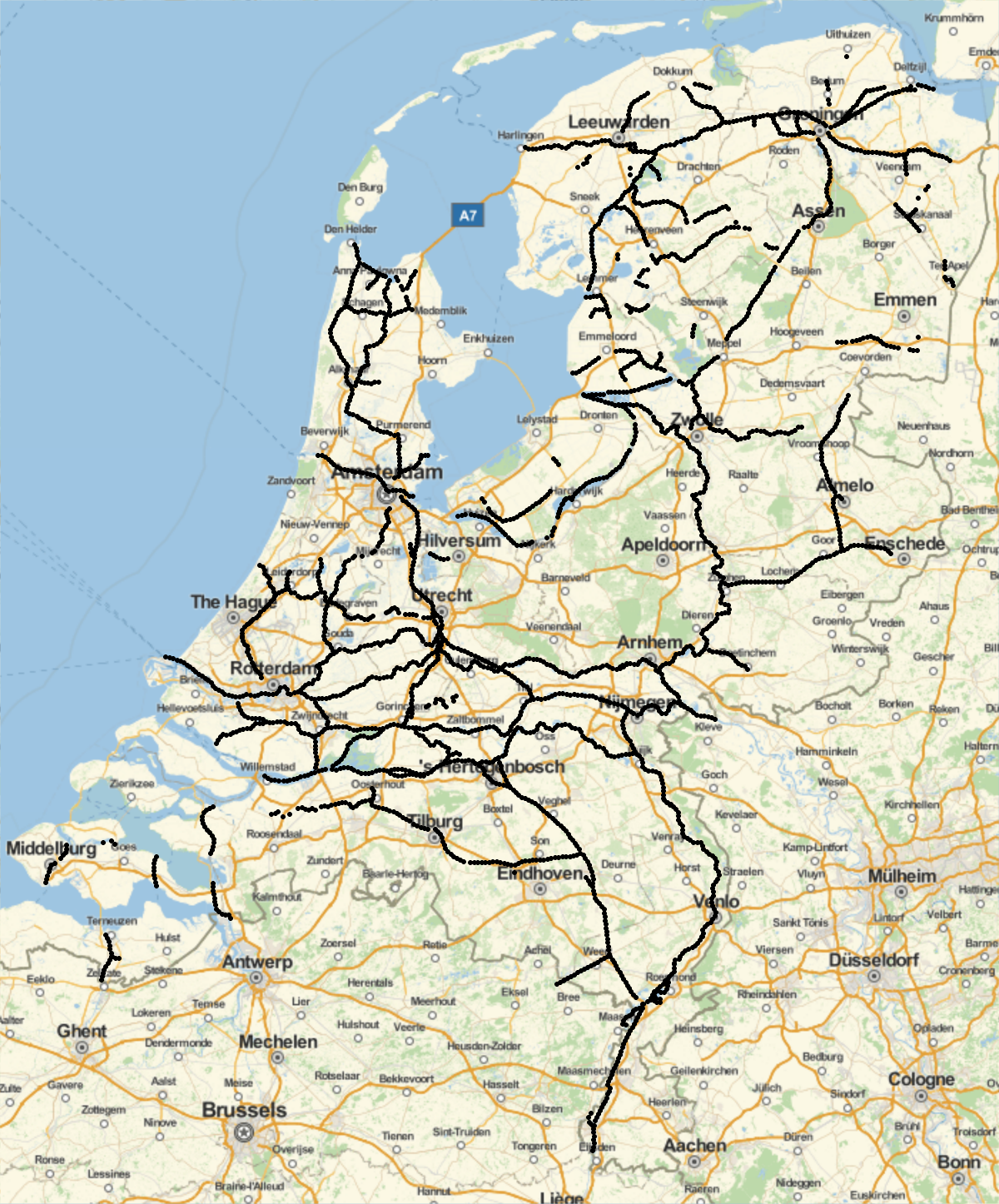### Properties and Relations

Using FromRDCoordinates and ToRDCoordinates, convert from and to RD coordinates:

 In:=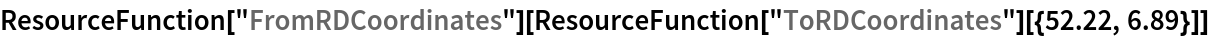Out=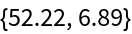The "origin" of the RD coordinates is somewhere in France:

 In:=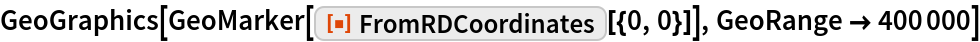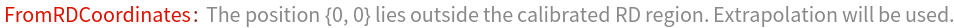Out=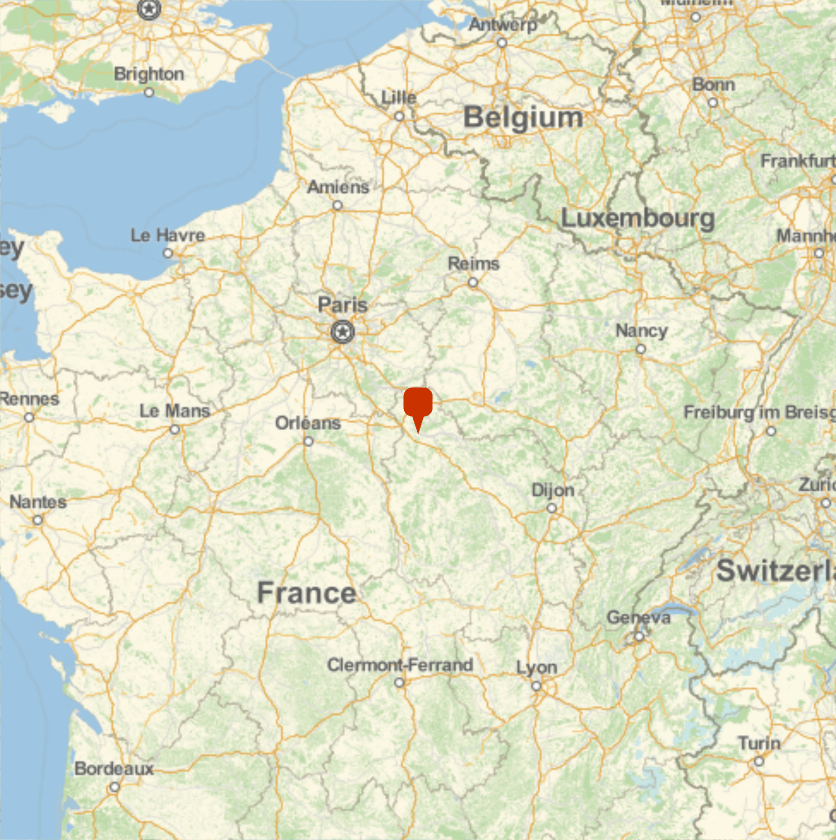The closest city is La Celle-Saint-Cyr:

 In:=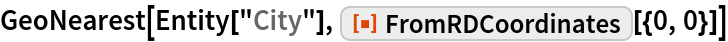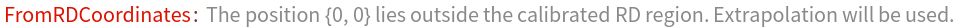Out=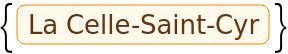### Possible Issues

The coordinates are valid only within the border given by the following RD coordinates:

 In:=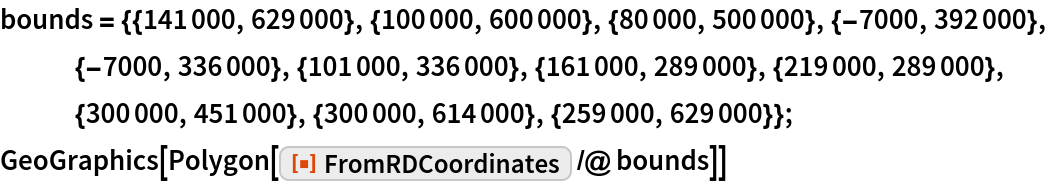Out=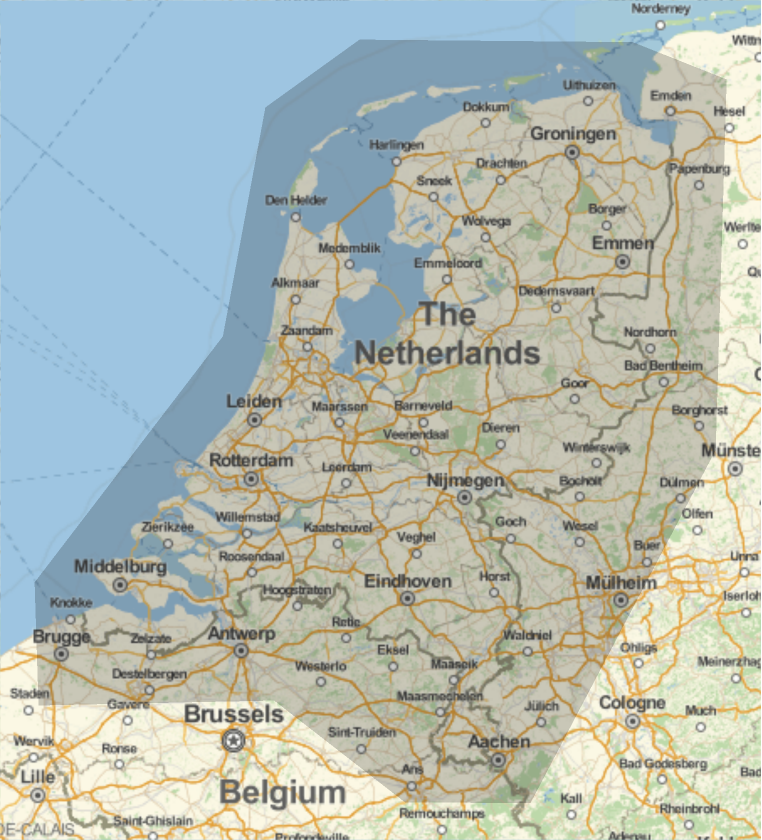Asking for a coordinate outside this region—in this example, Brussels—spawns a message and extrapolates the fit:

 In:=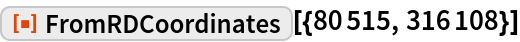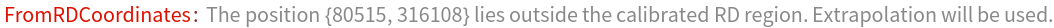Out=